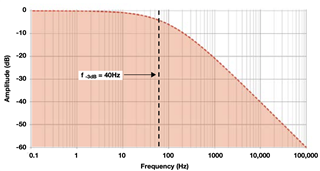If you have a related question, please click the "Ask a related question" button in the top right corner. The newly created question will be automatically linked to this question.

# ADS124S06: 3dB-Point at sampling rate?

Hi,

When I'm selecting any sampling rate from the ADC in combination with an sinc3 or LL filter is the output signal at the 3dB-point or without any signal strenght loss?

Thanks for helping.

Christian

• Hi Christian,

I'm not exactly sure what you are asking for, so I will answer the best I can.  The analog input signal is converted to a Delta-Sigma modulator bit stream, and the digital filter is basically a lowpass filter that filters quantization noise that has been pushed into higher frequencies during the oversampling/modulator process.  Any external frequencies of the input signal will also be filtered.  For example, power line-cycle frequencies would also be filtered at 50 and 60 Hz when using the data rate of 10sps.  So the digital filter will filter both the quantization noise and the analog input signal.

If you have a frequency of interest that is beyond the data rate selected, then you would need to use a faster data rate to capture that frequency.

Best regards,

Bob B

• Hi Bob,

thanks for the fast response, but it is still not clear for me.

For my understanding, all filters has some characteristics and are mostly defined by the cut off frequency (at -3db) in the frequency response curve, like here:Is this assumption also valid for the sinc and LL filters?

When I select a sample rate of the ADC, this defines also the maximum frequency. In combination with a sinc or LL filter, does the sample rate specify the -3db cut off frequency or is the max frequency for the selected sample rate before the amplitude decreases.

For example: Sampling rate is 1000SPS, Filter is sinc3 -> Max Frequency able to record is 1kHz -> Is the amplitude of an 1kHz-signal at the -3dB-Point?

In my application I need the full amplitude over the whole frequency defined by the sampling rate.

Best regards,

Christian

• Hi Christian,

The ADS124S06 device is a precision ADC where the sensor output is primarily dc, close to dc, or very slow moving.

All lowpass filters will have a cutoff frequency.  Depending on the type of filter the roll off response may be sharper than 20dB per decade.  The filtering characteristics for each filter type and data rate are shown in graphs starting on page 39 of the ADS124S06 datasheet.  Table 12 shows the -3dB cutoff frequency for each of the data rates using the low latency filter.  A similar sinc3 filter cutoff is shown in Table 14.

For your example, with sinc3 and 1000sps, the cutoff frequency as shown in Table 14 is 262 Hz.  If you need to capture 1000Hz you would need to use the 4ksps setting for sinc3 which has a filter cutoff of 1046 Hz.

Best regards,

Bob B